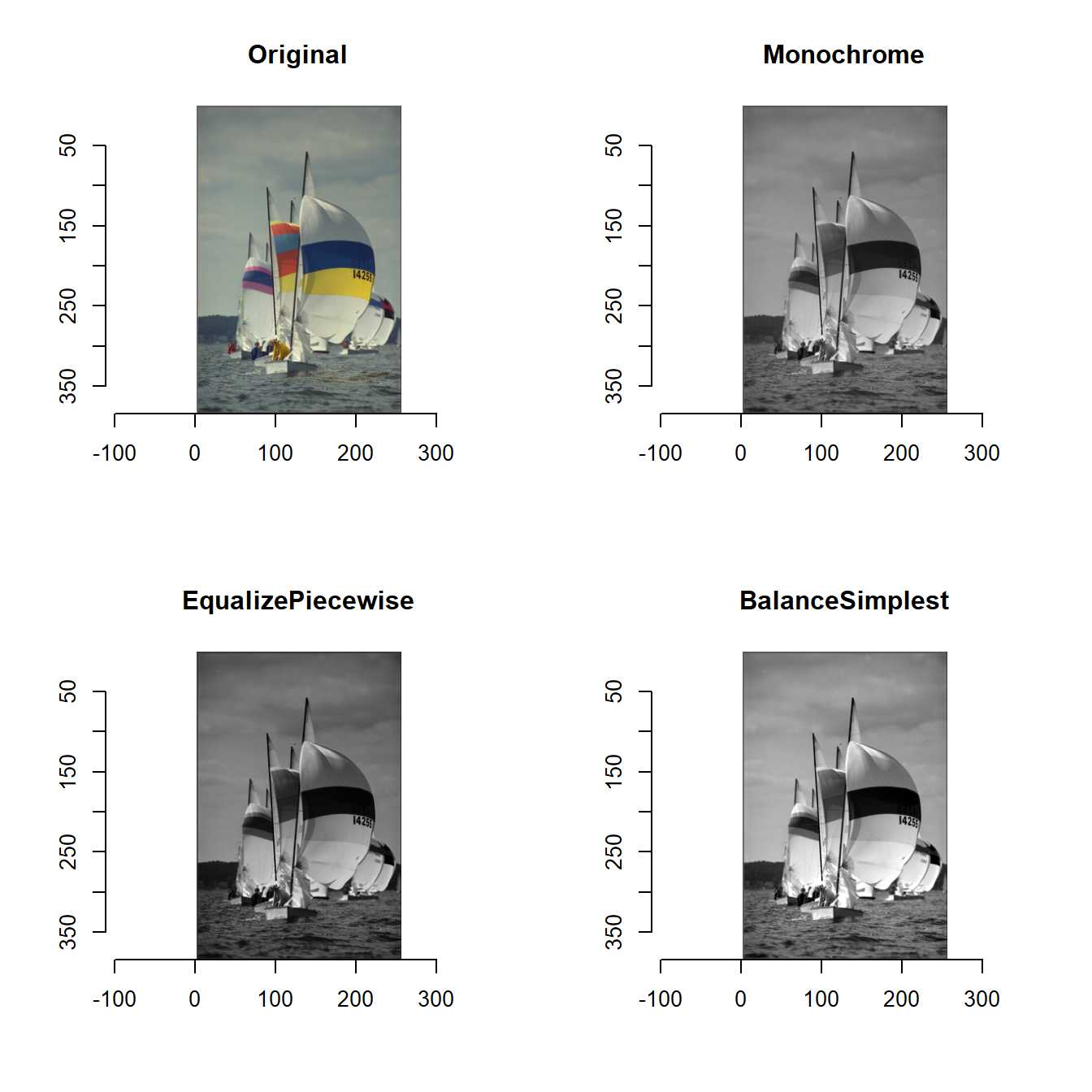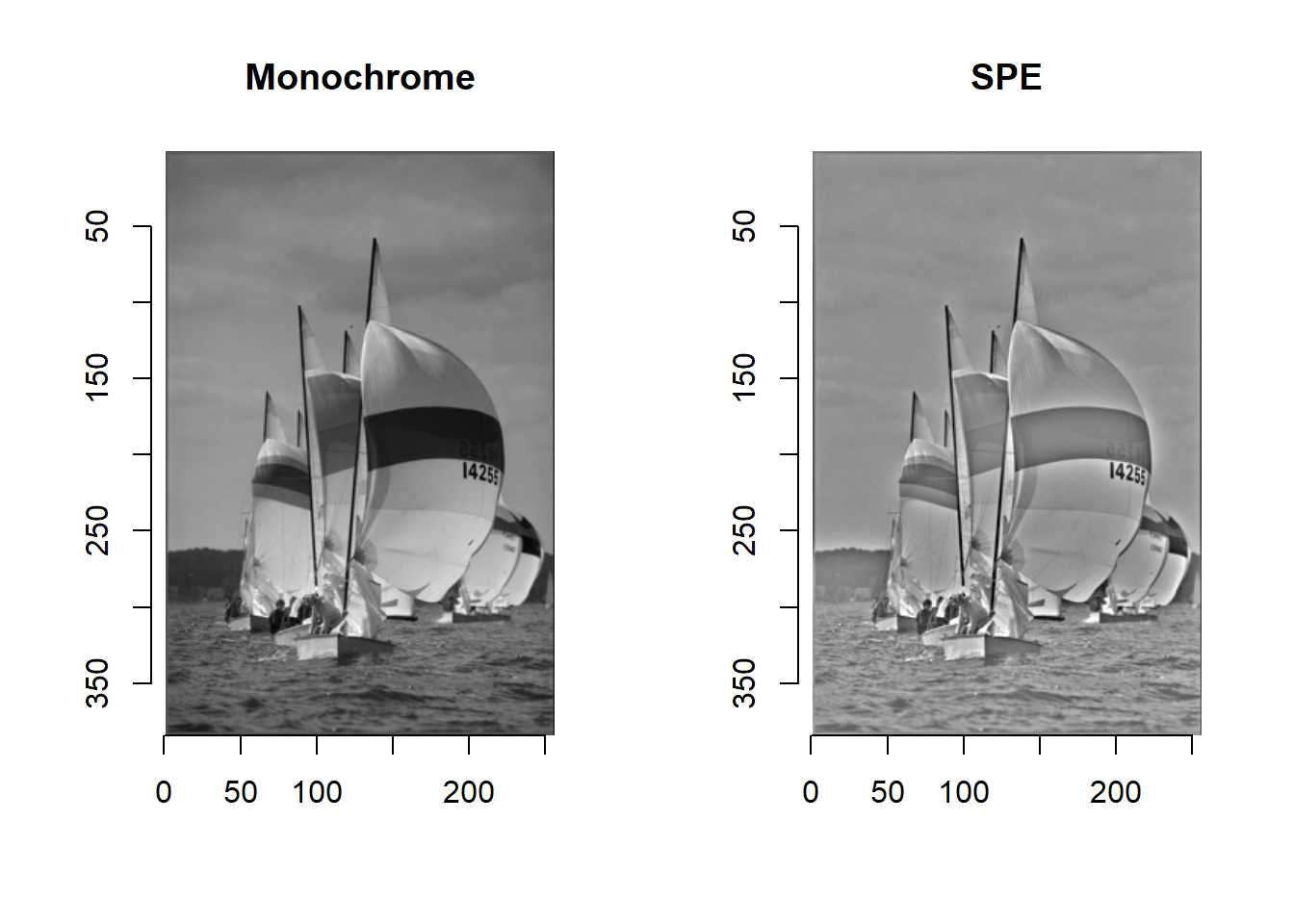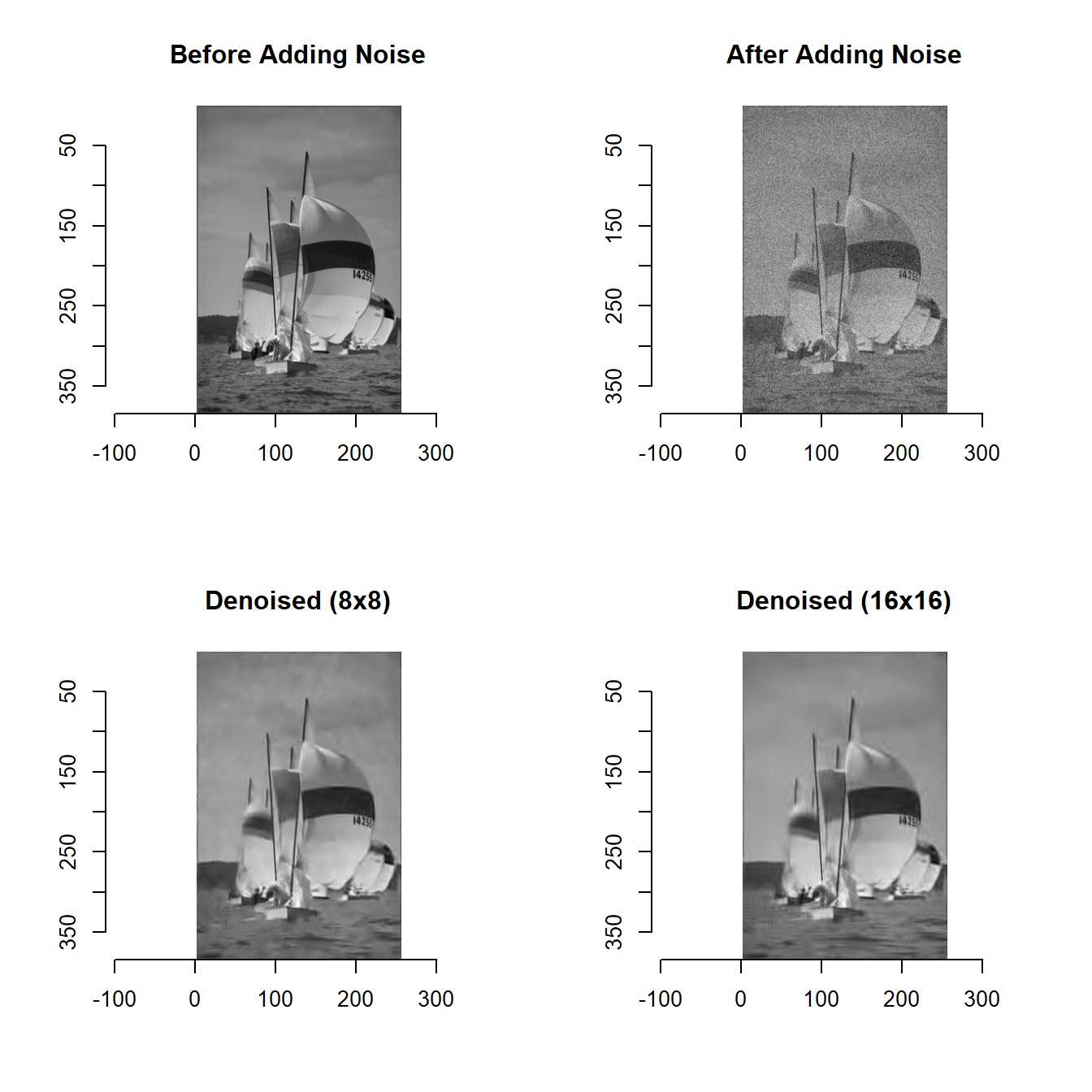announcement_imagerExtra.utf8

imagerExtra provides several advanced functions for image processing.

imagerExtra have four functions: EqualizePiecewise, BalanceSimplest, SPE, and DenoiseDCT.

EqualizePiecewise and BalanceSimplest enhance contrast of image.

library(imagerExtra)
g <- grayscale(boats)
layout(matrix(1:4, 2, 2, byrow = TRUE))
plot(boats, main = "Original")
plot(g, main = "Monochrome")
EqualizePiecewise(g, 100) %>% plot(main = "EqualizePiecewise")
BalanceSimplest(g, 1, 1) %>% plot(main = "BalanceSimplest")SPE corrects inhomogeneous background of image. Note that SPE take a lot of time because of the inefficient implementation of 2D DCT.

layout(matrix(1:2, 1, 2))
plot(g, main = "Monochrome")
SPE(g, 0.1) %>% plot(main = "SPE")DenoiseDCT denoises noise of image.

layout(matrix(1:4, 2, 2, byrow = TRUE))
noisy <- g + imnoise(sd = 0.1, dim = dim(g))
plot(g, main = "Before Adding Noise")
plot(noisy, main = "After Adding Noise")
DenoiseDCT(noisy, sdn = 0.1, flag_dct16x16 = FALSE) %>% plot(main = "Denoised (8x8)")
DenoiseDCT(noisy, sdn = 0.1, flag_dct16x16 = TRUE) %>% plot(main = "Denoised (16x16)")